Courses

# JIPMER Chemistry Mock Test - 3

## 60 Questions MCQ Test JIPMER: Subject wise Tests & Practice Mock Tests | JIPMER Chemistry Mock Test - 3

Description
This mock test of JIPMER Chemistry Mock Test - 3 for NEET helps you for every NEET entrance exam. This contains 60 Multiple Choice Questions for NEET JIPMER Chemistry Mock Test - 3 (mcq) to study with solutions a complete question bank. The solved questions answers in this JIPMER Chemistry Mock Test - 3 quiz give you a good mix of easy questions and tough questions. NEET students definitely take this JIPMER Chemistry Mock Test - 3 exercise for a better result in the exam. You can find other JIPMER Chemistry Mock Test - 3 extra questions, long questions & short questions for NEET on EduRev as well by searching above.
QUESTION: 1

Solution:
QUESTION: 2

Solution:
QUESTION: 3

### Acetylene gives

Solution:
QUESTION: 4

Which one of the following is not an isoelectronic pair

Solution:
QUESTION: 5

The first emission line in the atomic spectrum of hydrogen in the Balmer series appears at

Solution:
QUESTION: 6

The atomic number of an element is 35. What is the total number of electrons present in all the p-orbitals of the ground state atom of the element

Solution:
QUESTION: 7

The homologue of ethyne is

Solution:

C2H2 is Ethyne (homologue of alkyne)
C3H4 is propyne (homologue of alkyne)
C3H8 → propane (homologue of alkane)
C2H6 → Ethane (homologue of alkane)

QUESTION: 8

A one litre flask is full of brown bromine fumes. The intensity of brown colour of vapour will not decrease appreciably on adding to the flask some

Solution:
QUESTION: 9

The hybrid state of I in IF₅ is

Solution:
QUESTION: 10

Which is most ionic?

Solution:
QUESTION: 11

For a given reaction t1/2 = (1/ka). The order of reaction is

Solution:
QUESTION: 12

In a reversible reaction, the catalyst

Solution:
QUESTION: 13

The law formulated by Nernst is

Solution:
QUESTION: 14

The compound with carbon uses only its sp3 hybrid orbitals for bond formation is

Solution:
QUESTION: 15

The bond dissociation energies of H₂,Cl₂ and HCl are 104, 58 and 103 kcal respectively. The enthalpy of formation of HCl gas will be

Solution:
QUESTION: 16

The free energy change (∆Go) is negative when

Solution:
QUESTION: 17

An ester used as medicine is

Solution:
QUESTION: 18

The IUPAC name of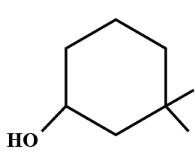is

Solution: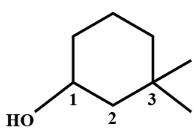QUESTION: 19

Which pair of atomic numbers represent elements which are both s-block elements

Solution:

Lithium (atomic number-3) is an alkali metal and magnesium (atomic number-12) is an alkaline earth metal.

QUESTION: 20

Which of the following is an example of a double salt?

Solution:
QUESTION: 21

What will be the e.m.f. of the given cell?
Pt | H2(P1)| H+(aq)|H2(P2)|Pt

Solution: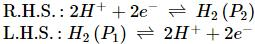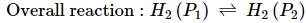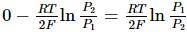QUESTION: 22

At which one of the following temperature-pressure conditions, the deviation of a gas from ideal behaviour is expected to be minimum ?

Solution:
QUESTION: 23

Cryolite is

Solution:
QUESTION: 24

300 ml of a gas at 27oC is cooled to -3oC at consatnt pressure, the final volume is

Solution:
QUESTION: 25

The strongest reducing agent of the alkali metal is

Solution:
QUESTION: 26

What is the molecular formula of Borazole?

Solution:
QUESTION: 27

Glass is a

Solution:
QUESTION: 28

C and Si have

Solution:
QUESTION: 29

What causes nitrogen to be chemically inert ?

Solution:
QUESTION: 30

What is the hydrization of S in SF₄ ?

Solution:
QUESTION: 31

Ozonised oxygen can be obtained from H₂O by the action of

Solution:
QUESTION: 32

Alkaline earth metals form ions of the formula

Solution:
QUESTION: 33

An acid solution of pH 6 is diluted hundred times. The pH of solution becomes approx.

Solution:
QUESTION: 34

When same amount of zinc is treated separately with excess of sulphuric acid and excess of sodium hydroxide solution the ratio of volumes of hydrogen evolved is

Solution:
QUESTION: 35

The Ksp of CuS,Ag₂S and HgS are 10⁻31, 10⁻44, 10⁻54 respectively.
The solubility of these sulphides are in the order

Solution: For CuS &HgS Ksp=S²..(where s= solubility) & for Ag2S ; Ksp=4S³(s= solubility) now put the values of Ksp and check in which case the value of S is highest & lowest.so.... the ans will be A
QUESTION: 36

FeCl₃ solution is

Solution:
QUESTION: 37

Isomers of propionic acid are

Solution:
QUESTION: 38

HCOOH reacts with conc.H₂SO₄ to produce

Solution:
QUESTION: 39

The decay constant of a radioactive sample is 'λ'. The half-life and mean life of the sample are respectively

Solution:
QUESTION: 40

The half life period of a radioactive material is 15 minutes. What percent of radioactivity of that material will remain after 45 minutes?

Solution: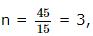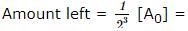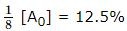QUESTION: 41

Which of the following compounds gives a ketone with Grignard reagent?

Solution:
QUESTION: 42

The reagent used to convert Ethanoic acid to Ethanol is

Solution:
QUESTION: 43

Which one of the following is reduced with Zn and HCl to give the corresponding hydrocarbon?

Solution:
QUESTION: 44

What is formed when a primary alcohol undergoes catalytic dehydrogenation?

Solution:
QUESTION: 45

Most stable alkene is :

Solution:
QUESTION: 46

Which of the following complexes is likely to show optical activity?

Solution:
QUESTION: 47

Which gives more stable product ?

Solution:
QUESTION: 48

Phenol is heated with CHCl₃ and alcoholic KOH when salicylaldehyde is produced. This reaction is known as

Solution:
QUESTION: 49

Ziegler-Natta catalyst is

Solution:
QUESTION: 50

On analysis ,a saturated hydrocarbon is found to contain 83.70% carbon and 16.30% hydrogen .The empirical formula will be (At.wt.of C = 12; At.wt.of H = 1) ?

Solution:

C : H ratio is 83.70/12 : 16.30/1 or 6.975 : 16.30 or 1 : 2.33 or 3 : 8.
Hence empirical formula is C3H8

QUESTION: 51

When iron or zinc is added to CuSO solution, copper is precipitated. It is due to

Solution:
QUESTION: 52

The oxidation number of Mn in KMnO₄ is

Solution:
QUESTION: 53

A f.c.c. crystal contains how many atoms in each unit cell?

Solution:
QUESTION: 54

At a given temperature, the vapour pressure of pure A and B is 108 and 36 torr respectively. What will be the mole fraction of B in the vapour phase whih is in equilibrium with a solution containing equimole fraction of A and B, under ideal behaviour conditions ?

Solution:
QUESTION: 55

Very dilute solutions which show deviations (positive or negative) from Raoult's law are called

Solution:
QUESTION: 56

Which of the following compounds can show optical isomerism?

Solution:
QUESTION: 57

A compound contains 28% nitrogen and 72% of a metal by weight.If 3 atoms of the metal combine with 2 atoms of nitrogen, then atomic weight of the metal is

Solution:
QUESTION: 58

The colloidal sols are purified by

Solution:
QUESTION: 59

The calculated magnetic moment (in Bohr magnetons) of Cu+2 ion is

Solution:
QUESTION: 60

When a copper wire is dipped into silver nitrate solution, the solution gradually becomes blue. The reason for this is

Solution:

Track your progress, build streaks, highlight & save important lessons and more!

### Similar Content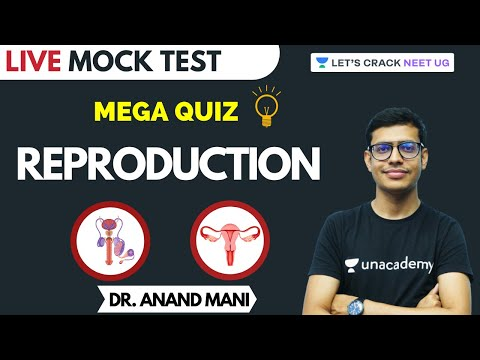### Related tests